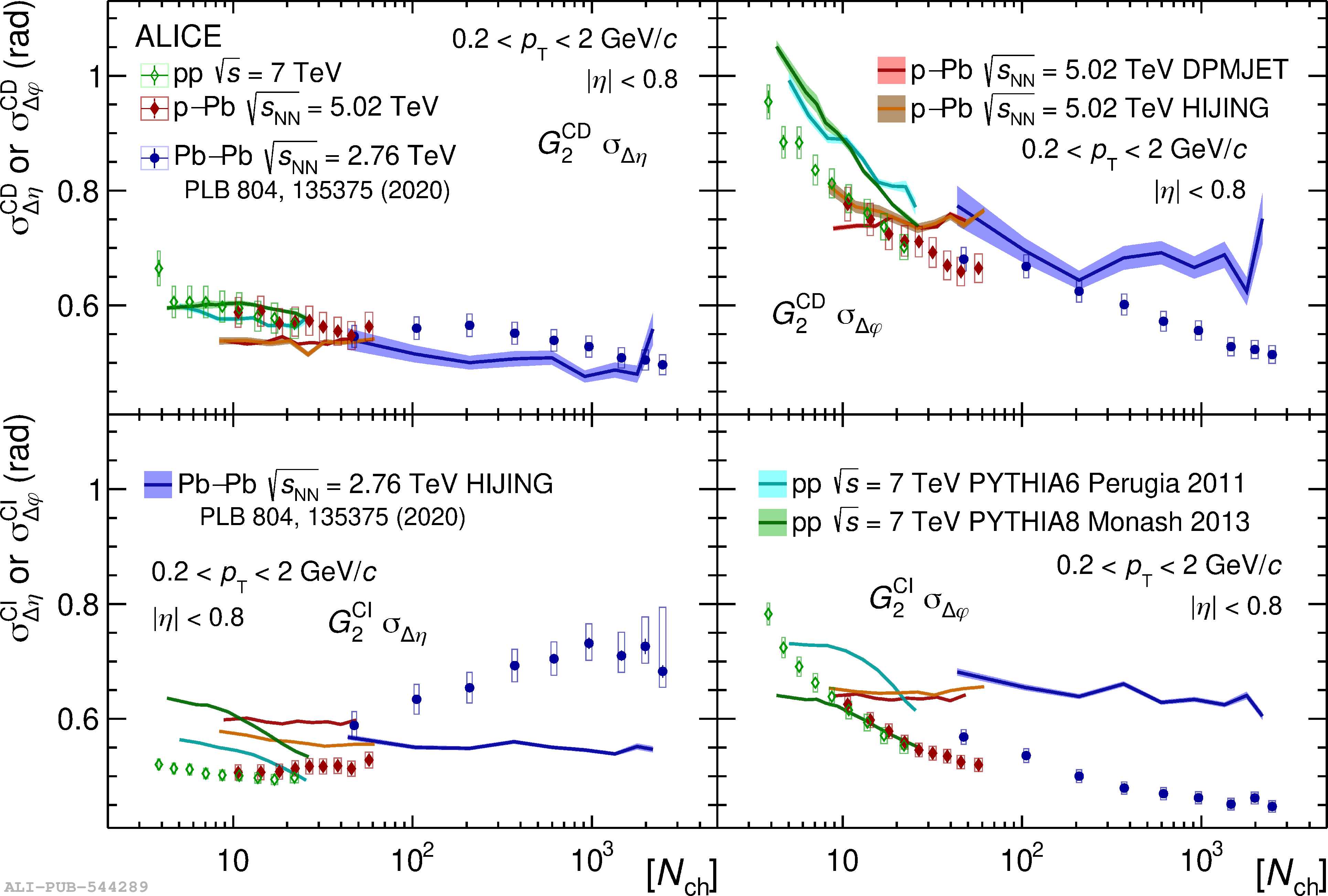# Figure 8

 Evolution with the average charged particle multiplicity of the longitudinal (left) and azimuthal (right) widths of the two-particle transverse momentum correlations $G_{2}^{\rm CD}$ (top row) and $G_{2}^{\rm CI}$ (bottom row) in pp, p--Pb, and Pb--Pb collisions at $\sqrt{s} = 7\;\text{TeV}$, $\sqrt{s_{\rm NN}} = 5.02\;\text{TeV}$, and $\sqrt{s_{\rm NN}} = 2.76\;\text{TeV}$, respectively, compared to models. Statistical and systematic uncertainties of the data points are shown as vertical bars (mostly smaller than the marker size) and filled boxes, respectively, while the thickness of the shaded bands represents statistical uncertainties of the models. The data points and the results of HIJING simulations for Pb--Pb collisions are taken from Ref. .